# streamslice

Plot streamlines in slice planes

•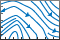## Syntax

``streamslice(X,Y,Z,U,V,W,xslice,yslice,zslice)``
``streamslice(U,V,W,xslice,yslice,zslice)``
``streamslice(X,Y,U,V)``
``streamslice(U,V)``
``streamslice(___,arrowsmode)``
``streamslice(___,density)``
``streamslice(___,method)``
``streamslice(ax,___)``
``lineobj = streamslice(___)``
``[verts,averts] = streamslice(___)``

## Description

example

````streamslice(X,Y,Z,U,V,W,xslice,yslice,zslice)` draws automatically spaced streamlines from 3-D vector data `U`, `V`, and `W`. Specify `X`, `Y`, and `Z` as the coordinate data. Specify `xslice`, `yslice`, and `zslice` as the slice locations orthogonal to a particular axis.```
````streamslice(U,V,W,xslice,yslice,zslice)` uses the default coordinate data for `U`, `V`, and `W`. The (x,y,z) location for each element in `U`, `V`, and `W` is based on the column, row, and page index, respectively. ```

example

````streamslice(X,Y,U,V)` draws automatically spaced streamlines from 2-D vector data `U` and `V`. Specify `X` and `Y` as the coordinate data.```

example

````streamslice(U,V)` uses the default coordinate data for `U` and `V`. The (x,y) location for each element in `U` and `V` is based on the column and row index, respectively.```

example

````streamslice(___,arrowsmode)`, where `arrowsmode` is `'noarrows'`, does not draw direction arrows. The default for `arrowsmode` is `'arrows'`, which draws direction arrows. Use this option with any of the input arguments from the previous syntaxes.```

example

````streamslice(___,density)` modifies the automatic spacing of the streamlines, where `density` is a positive scalar. The default value is `1`. Higher values produce more streamlines on each plane. For example, `2` produces approximately twice as many streamlines as the default, while `0.5` produces approximately half as many.```
````streamslice(___,method)` specifies the interpolation method, where method can be `'linear'`, `'cubic'`, or `'nearest'`.```
````streamslice(ax,___)` plots into the specified axes, instead of into the current axes object (`gca`). ```

example

````lineobj = streamslice(___)` returns the `Line` objects created.```

example

````[verts,averts] = streamslice(___)` returns vertices for drawing the streamlines and arrows as cell arrays. This syntax does not plot the streamlines.```

## Examples

collapse all

Load the `wind` dataset, which loads the 3-D arrays `u`, `v`, `w`, `x`, `y`, and `z`, representing air currents over regions of North America. Draw streamlines along the slice plane where `z = 5`.

```load wind streamslice(x,y,z,u,v,w,[],[],5) axis tight```Load the `wind` dataset and draw streamlines without direction arrows along the slice plane where `z = 5`.

```load wind streamslice(x,y,z,u,v,w,[],[],5,'noarrows') axis tight```Load the `wind` dataset and draw streamlines along the slice plane, where `z = 5`. Use a `density` value of `2` to draw approximately twice as many streamlines as the default.

```load wind streamslice(x,y,z,u,v,w,[],[],5,2) axis tight```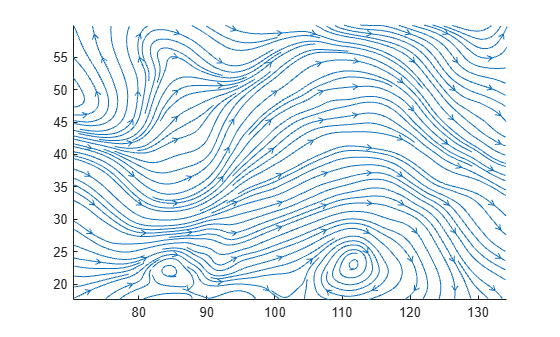Load the `wind` dataset and compute the vertices of streamlines along the slice planes, where `z = 1`, 5, and 10.

```load wind [verts, ~] = streamslice(x,y,z,u,v,w,[],[],[1,5,10]);```

Pass the vertices to `streamtube` to visualize the lines as tubes.

```streamtube(verts,1) view(3) axis tight shading interp camlight lighting gouraud```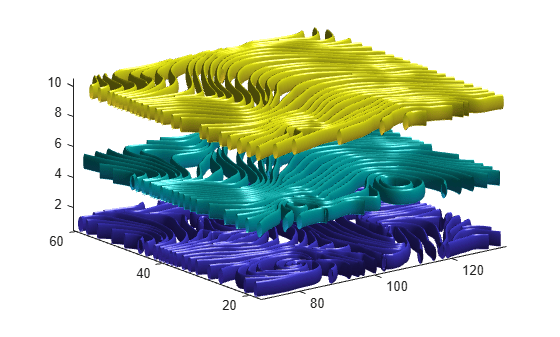Plot streamlines and store the returned `Line` objects in `l`.

```[x,y] = meshgrid(-10:10); u = 2.*x.*y; v = y.^2 - x.^2; l = streamslice(x,y,u,v); axis tight```Set the line width of the streamlines to 2. Set their color to red. Use the `set` function to change the properties of all the streamlines at the same time.

```set(l,'LineWidth',2) set(l,'Color','r');```First, create a surface plot.

```z = peaks; surf(z); shading interp;```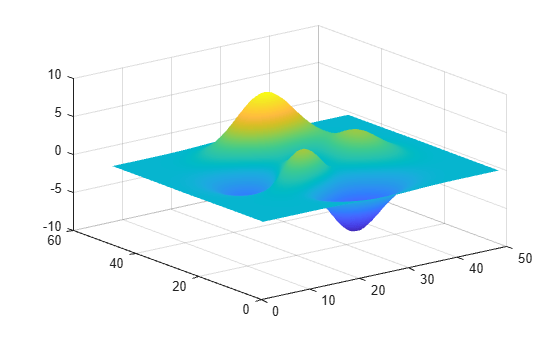Compute the two-dimensional gradient of `z`. Use the `x` and `y` components as the vector data input for `streamslice`. Set the color of the streamlines to black.

```[u,v] = gradient(z); l = streamslice(u,v); set(l,'Color','k');```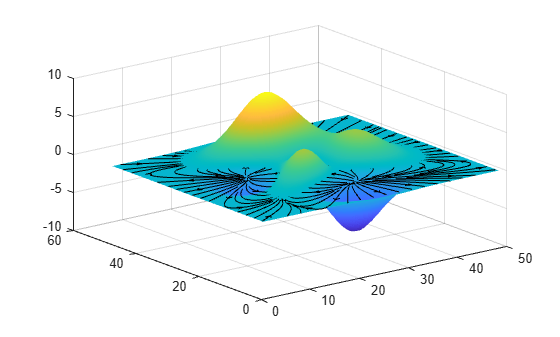Displace the streamlines on the `z`-axis so that they are drawn on the surface of the curve.

```for i=1:length(l) zi = interp2(z,l(i).XData, l(i).YData); l(i).ZData = zi; end```## Input Arguments

collapse all

x-axis coordinate data, specified as a 2-D or 3-D array. It must be monotonic, but does not need to be uniformly spaced.

`X`, `Y`, `Z`, `U`, `V`, and `W` must be the same size.

You can use the `meshgrid` function to create `X`, `Y`, and `Z` matrices.

y-axis coordinate data, specified as a 2-D or 3-D array. It must be monotonic, but does not need to be uniformly spaced.

`X`, `Y`, `Z`, `U`, `V`, and `W` must be the same size.

You can use the `meshgrid` function to create `X`, `Y`, and `Z` matrices.

z-axis coordinate data, specified as a 3-D array. It must be monotonic, but does not need to be uniformly spaced.

`X`, `Y`, `Z`, `U`, `V`, and `W` must be the same size.

You can use the `meshgrid` function to create `X`, `Y`, and `Z` matrices.

x-components of vector data, specified as a 2-D or 3-D array.

`X`, `Y`, `Z`, `U`, `V`, and `W` must be the same size.

y-components of vector data, specified as a 2-D or 3-D array.

`X`, `Y`, `Z`, `U`, `V`, and `W` must be the same size.

z-components of vector data, specified as a 3-D array.

`X`, `Y`, `Z`, `U`, `V`, and `W` must be the same size.

x-axis slice values, specified in one of these forms:

• Scalar — Draw one slice plane orthogonal to the x-axis at the specified location.

• Vector — Draw multiple slice planes orthogonal to the x-axis at the specified locations.

• [] — Do not draw any slice planes.

y-axis slice values, specified in one of these forms:

• Scalar — Draw one slice plane orthogonal to the y-axis at the specified location.

• Vector — Draw multiple slice planes orthogonal to the y-axis at the specified locations.

• [] — Do not draw any slice planes.

z-axis slice values, specified in one of these forms:

• Scalar — Draw one slice plane orthogonal to the z-axis at the specified location.

• Vector — Draw multiple slice planes orthogonal to the z-axis at the specified locations.

• [] — Do not draw any slice planes.

Streamline density, specified as a positive scalar.

The default value is `1`. Higher values produce more streamlines on each plane. For example, `2` produces approximately twice as many streamlines as the default, while `0.5` produces approximately half as many.

Arrow display mode, specified as one of these values:

• `'arrows'` — Draw direction arrows on the streamlines.

• `'noarrows'` — Do not draw direction arrows on the streamlines.

Interpolation method, specified as one of these values:

• `'linear'` — Linear interpolation of the values at neighboring grid points in each respective dimension.

• `'cubic'` — Cubic interpolation of the values at neighboring grid points in each respective dimension.

• `'nearest'` — Nearest grid point value.

Target axes, specified as an `Axes` object. If you do not specify the axes, then `streamslice` plots into the current axes.

## Output Arguments

collapse all

`Line` objects for streamlines and direction arrows, returned as an array.

Streamline vertices, returned as a cell array. Each element of the cell array is a matrix of vertices for one line.

You can pass these values to any of the streamline drawing functions (`streamline`, `streamribbon`, `streamtube`).

Arrow vertices, returned as a cell array. Each element of the cell array is a matrix of vertices for one arrow. If `arrowsmode` is `'noarrows'`, averts is an empty cell array.

You can pass these values to `streamline`.

## Version History

Introduced before R2006a